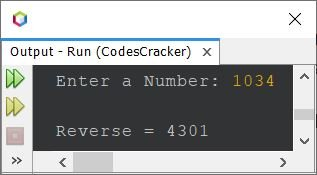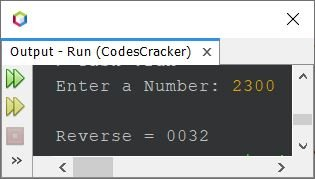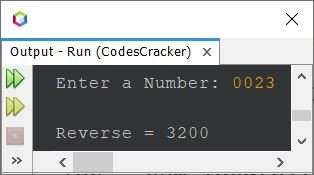# Java Program to Reverse a Number

This article covers a program in Java that find and prints the reverse of a number. For example, if user enters a number say 123, then the output will be 321.

## Reverse a Number in Java using while Loop

The question is, write a Java program to reverse a number. The number to reverse, must be received by user at run-time of the program. The answer to this question, is following program:

```import java.util.Scanner;

public class CodesCracker
{
public static void main(String[] args)
{
int num, rem, rev=0;
Scanner s = new Scanner(System.in);

System.out.print("Enter a Number: ");
num = s.nextInt();

while(num!=0)
{
rem = num%10;
rev = (rev*10) + rem;
num = num/10;
}

System.out.println("\nReverse = " +rev);
}
}```

The snapshot given below shows the sample run of above Java program, with user input 1034 as number to reverse:## Reverse a Number in Java using for Loop

Since the above program uses while loop, to reverse a given number. Therefore I've modified that program and created a new one, using for loop:

```import java.util.Scanner;

public class CodesCracker
{
public static void main(String[] args)
{
int num, rem, rev;
Scanner s = new Scanner(System.in);

System.out.print("Enter a Number: ");
num = s.nextInt();

for(rev=0; num!=0; num=num/10)
{
rem = num%10;
rev = (rev*10) + rem;
}

System.out.println("\nReverse = " +rev);
}
}```

This program produces exactly same output as of previous program. The following two statements, from above program:

```rem = num%10;
rev = (rev*10) + rem;```

can be replaced with a single statement, given below:

`rev = (rev*10) + (num%10);`

## Reverse a Number in Java using Array

This program uses array to do the same job as of previous program.

```import java.util.Scanner;

public class CodesCracker
{
public static void main(String[] args)
{
int num, len, i;
Scanner s = new Scanner(System.in);

System.out.print("Enter a Number: ");
num = s.nextInt();

len = String.valueOf(num).length();
int[] arr = new int[len];
for(i=0; i<len; i++)
{
arr[i] = num%10;
num = num/10;
}

System.out.print("\nReverse = ");
for(i=0; i<len; i++)
System.out.print(arr[i]);
}
}```

Since above program has a limitation. The limitation is, when user enters a number say 1230 or 03201. That is, 0 as the last or the first digit of a number. In that case, that 0 will get removed. As 013 is equal to 13. Therefore, I've created another version of the program, to remove this limitation. That program is given below, in the complete version.

## Reverse a Number in Java - Complete Version

I'm saying this program as a complete version of the reversing a number program in Java, because this program also produces the exact reverse of a number ending or starting with 0. For example, reverse of 00134 will be 43100. similarly reverse of 120 will be 021.

```import java.util.Scanner;

public class CodesCracker
{
public static void main(String[] args)
{
int len, i;
String num;
Scanner s = new Scanner(System.in);

System.out.print("Enter a Number: ");
num = s.next();

len = num.length();
char[] arr = new char[len];
for(i=0; i<len; i++)
arr[i] = num.charAt(i);

System.out.print("\nReverse = ");
for(i=(len-1); i>=0; i--)
System.out.print(arr[i]);
}
}```

Here is its sample run with user input 2300 as number to reverse:Here is another sample run with user input 0023#### Same Program in Other Languages

Java Online Test

« Previous Program Next Program »